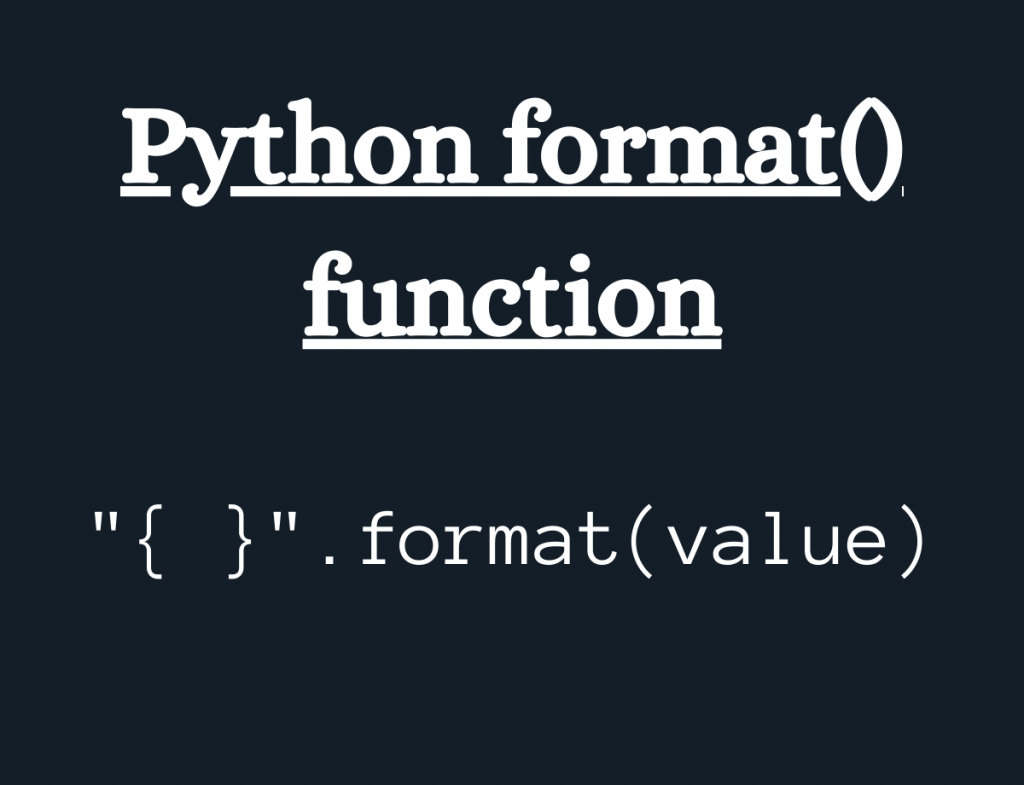# Python format() functionHello! In this article, we will be focusing on formatting string and values using Python format() function.

## Getting started with the Python format() function

Python format() function is an in-built String function used for the purpose of formatting of strings.

The Python `format() function` formats strings according to the position. Thus, the user can alter the position of the string in the output using the format() function.

Syntax:

```"{}".format(value)
```
• `{}`: These curly braces act as a formatter and when the function is called they are replaced with the string to be placed at the defined position.
• `value`: This parameter can be a string or a number or even a floating point integer. It represents the value to be replaced by the formatter in the output.

Example:

```s1 = 'Python'
s2 = 'with'
s4 = 'JournalDev'

s3 = "{} {} {}".format(s1, s2, s4)
print(s3)
```

Output:

```Python with JournalDev
```

## Index formatting with Python format()

The format() function also serves the purpose of formatting string at user defined positions i.e. we can change the position of the string or value to be placed in the output by specifying the index values inside the curly braces.

Example:

```s1 = 'Python'
s2 = 'with'
s4 = 'Data Science'

res = "{2} {1} {0}".format(s1, s2, s4)
print(res)
```

In the above snippet of code, the format(s1, s2, s4) internally assigns them index values as 0, 1, 2, and so on to the values passed to the function.

That is, s1 is assigned to index 0, s2 is assigned to index 1 and s4 is assigned to index 2.

So, by passing the index value of the string in the {}, we can alter the position of the string by the index values.

Output:

```Data Science with Python
```

## Passing values to the arguments in format() function

Using Python format() function, we can assign values to the variables we want to be displayed inside the parameter list of the function itself.

Syntax:

```"{var1}".format(var1='value')
```

Example:

```res = "{s4} {s2} {s1}".format(s1 = 'Python',s2 = 'with',s4 = 'Data Science')
print(res)
```

Output:

```Data Science with Python
```

## Padding a formatted string with Python format() function

The strings can also be formatted in terms of the alignment and padding using the format() function.

Syntax:

```#left padding
"{:>number}".format(value)
"{:<number}".format(value)
"{:^number}".format(value)
```

By passing a particular number, it serves as the number of characters to be padded around the string.

Example:

## Passing dict as parameter to Python format() function

Python Dictionary can also be passed to format() function as a value to be formatted.

Syntax:

```"{key}".format(**dict)
```

The key is passed to the {} and the format() function is used to replace the key by it’s value, respectively.

Since, we are passing the dict keys as arguments, in order to replace the keys with their values we need to unpack the dictionary. Thus, `Python "**" operator` is used to unpack the dictionary.

Example:

```dict1 = {"Python":"A","Java":"B","Cpp":"D"}
res = "{Python} {Cpp}".format(**dict1)
print(res)
```

Output:

```A D
```

## Passing complex number as an argument to the format() function

Python format() function can be used to access the Real and the Imaginary values from a complex number.

Syntax:

```"{0.real},{0.imag}".format(complex_number)
```

Example:

```num = 20-3j
res = "Real-part:{0.real}, Imaginary-part:{0.imag}".format(num)
print(res)
```

Output:

```Real-part:20.0, Imaginary-part:-3.0
```

## Python format() function using comma as separator value

Python format() function enables us to separate the numeric values using `"," as a separator`.

Syntax:

```"{:,}".format(number)
```

The separator i.e. “,” is added after three digits in a manner that it works for the thousands place in the number system.

Example:

```num = 10000000000
res = "{:,}".format(num)
print(res)
```

Output:

```10,000,000,000
```

## Formatting numbers using format() function

Python format() function has the feature of formatting numbers too.

In addition to formatting the numeric values, the format() function can be used to represent the numeric values according to the different number systems such as binary, octal, hexadecimal, etc.

Syntax:

```#Hexadecimal representation
"{0:x}".format(value)
#Octal representation
"{0:o}".format(value)
#Decimal representation
"{0:d}".format(value)
#Binary representation
"{0:b}".format(value)
```

Example 1:

```num = 10
binary = "{0:b}".format(num)
print("Binary representation:", binary)
octal = "{0:o}".format(num)
print("Octal Decimal representation:", octal)
decimal = "{0:d}".format(num)
print("Decimal representation:", decimal)
```

Output:

```Binary representation: 1010
Octal Decimal representation: 12
Decimal representation: 10
```

## Conclusion

Thus, in this article, we have looked at the working of Python format() function along with the various uses of the function with strings, numbers, etc.

To know more about formatting of Strings, I would strongly recommend the readers to learn about Python f-string.

## References

• Python format() function — JournalDev